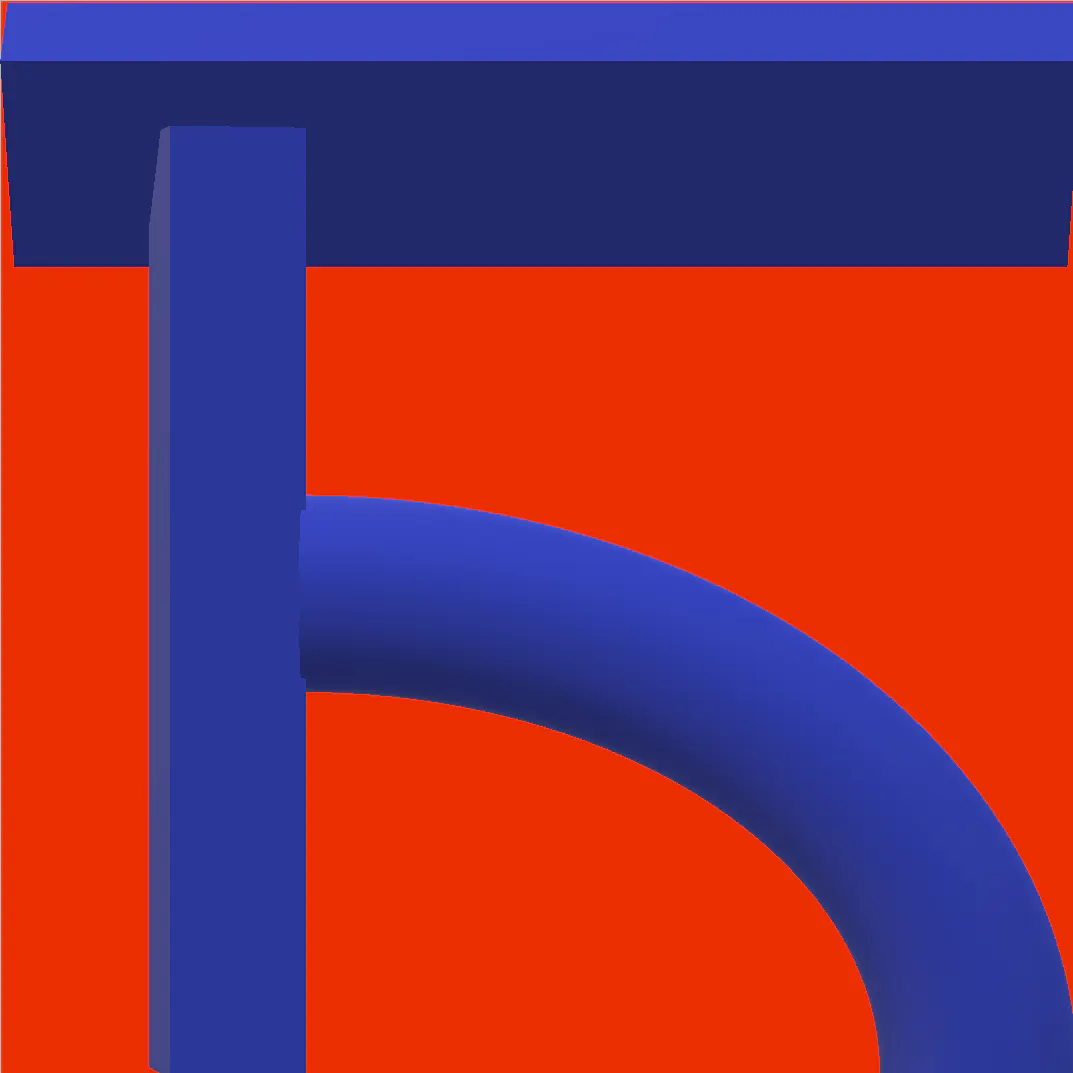# Nano Weather Shield V1.1

Display weather information and activate RGB color patterns via this shield, including built-in BMP280 and photoresistor, designed by me.

ExpertFull instructions provided2 hours1,501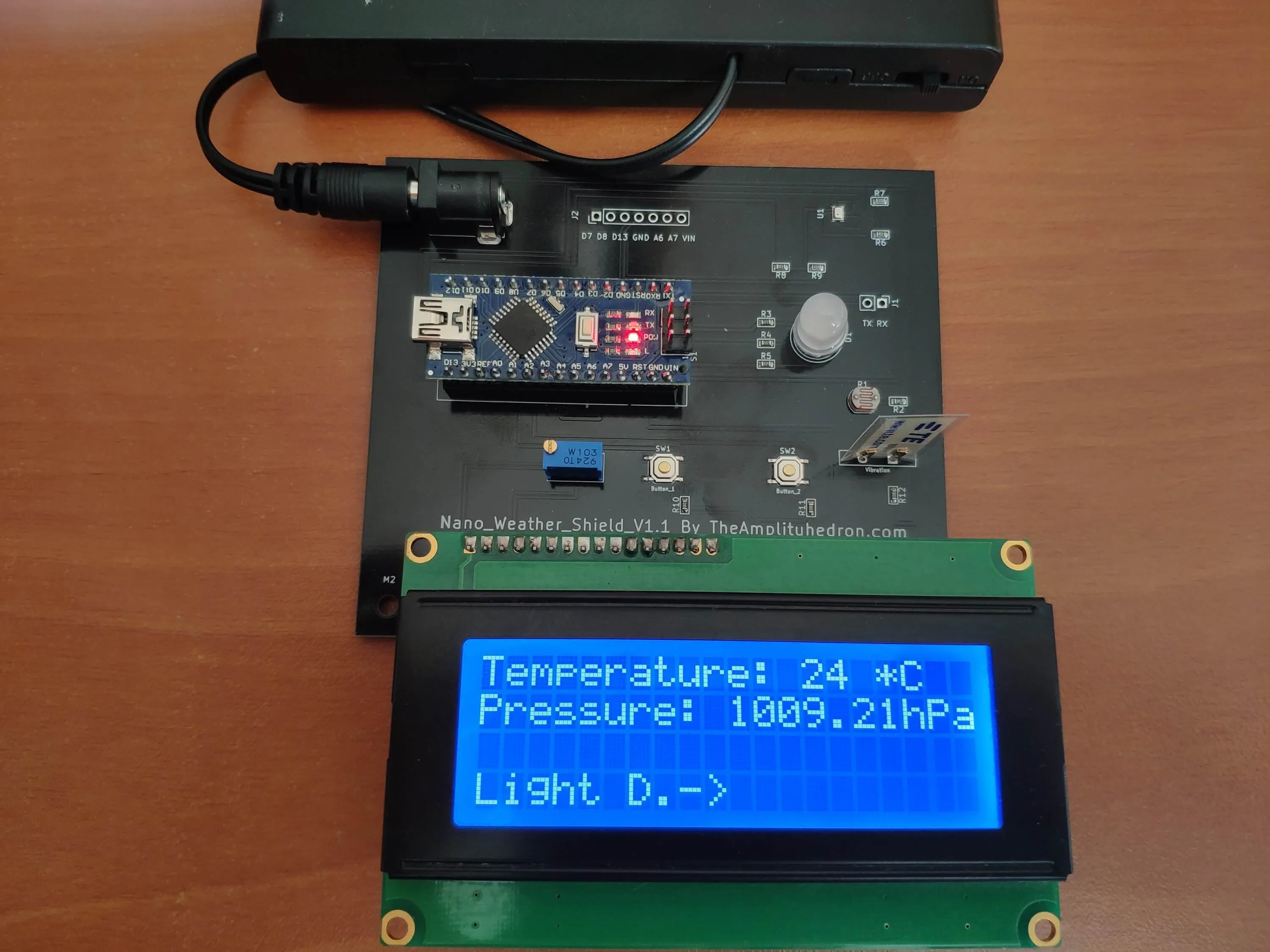## Things used in this project

### Hardware components

 Seeed Nano Weather Shield V1.1
×1Arduino Nano R3
×1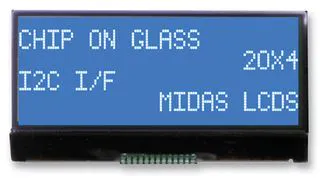Alphanumeric LCD, 20 x 4
×1

### Software apps and online servicesArduino IDE## Schematics

### Eeschema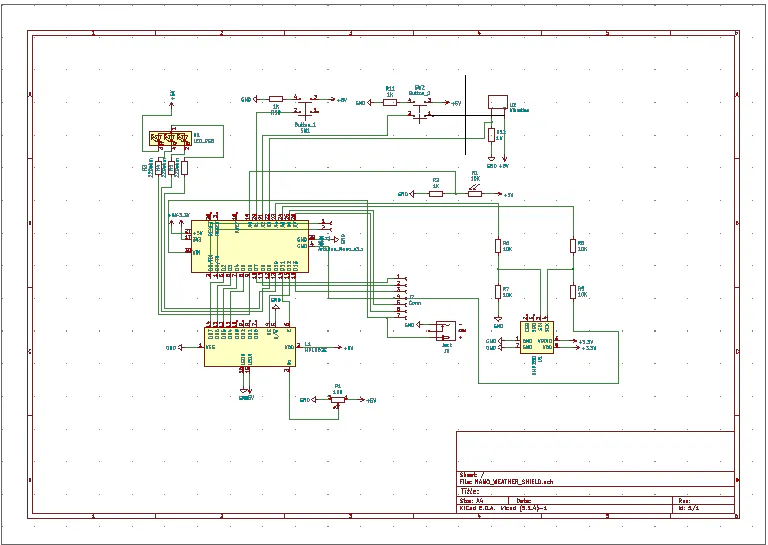### Footprint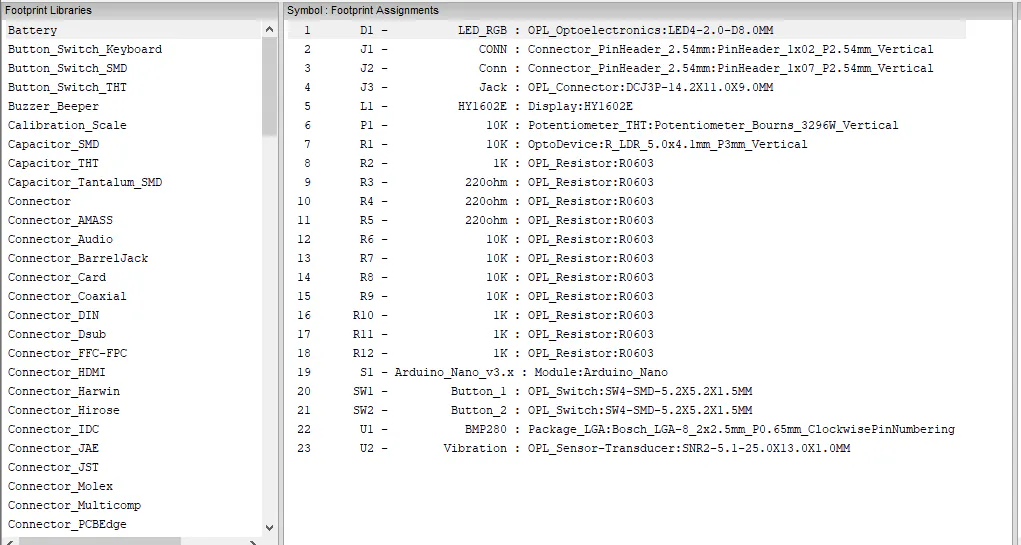### Pcbnew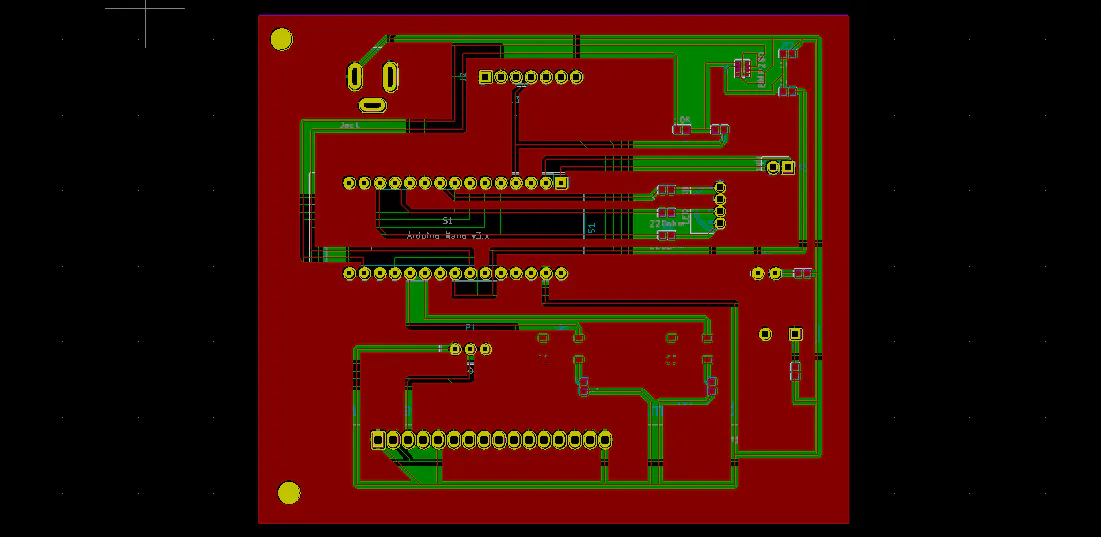## Code

### Arduino Nano Code

Arduino
```         /////////////////////////////////////////////
//       Nano_Weather_Shield_V1.1          //
//                                         //
//      -----------------------------      //
//             (Arduino Nano)              //
//             by Kutluhan Aktar           //
//                                         //
/////////////////////////////////////////////

// Following code is for Nano Weather Shield V1.1 by TheAmplituhedron.
//
// https://www.theamplituhedron.com/projects/Nano-Weather-Shield-V1.1/
//
// Connections
// Nano_Weather_Shield_V1.1 :
//                                16x2 or 20x4 LCD Screen
// D12 --------------------------- rs
// D11 --------------------------- en
// D5  --------------------------- D4
// D4  --------------------------- D5
// D3  --------------------------- D6
// D2  --------------------------- D7
//                                RGB LED
// D6  --------------------------- r
// D9  --------------------------- g
// D10 --------------------------- b
//                                BMP280 Pressure and Temperature Sensor
// A4 --------------------------- SDI
// A5 --------------------------- SCK
//                                Vibration Sensor
// A3 --------------------------- S
//                                Photoresistor
// A0 --------------------------- S
//                                Button_1
// A1 ---------------------------
//                                Button_2
// A2 ---------------------------

// include the required libraries for the BMP280 Temperature and Pressure Sensor
#include <Wire.h>

// include the LiquidCrystal library:
#include <LiquidCrystal.h>

// initialize the library by associating any needed LCD interface pin
// with the arduino pin number it is connected to
const int rs = 12, en = 11, d4 = 5, d5 = 4, d6 = 3, d7 = 2;
LiquidCrystal lcd(rs, en, d4, d5, d6, d7);

// define sensors' signal pins.
#define Photo_Pin A0
#define Vibra_Pin A3
//define RGB pins
#define R 6
#define G 9
#define B 10
// define button pins
#define button_1 A1
#define button_2 A2

// define screen booleans so as to switch between screens - SCREEN_2 is the home screen.
boolean SCREEN_1 = true;
boolean SCREEN_2 = false;
boolean SCREEN_3 = false;

// define data holders
int swb_1, swb_2, photoresistor, vibration;
float temperature, pressure;
int screen_number = 0;

void setup() {
Serial.begin(9600);

pinMode(R, OUTPUT);
pinMode(G, OUTPUT);
pinMode(B, OUTPUT);
analogWrite(R, 255);
analogWrite(G, 255);
analogWrite(B, 255);

// set up the LCD's number of columns and rows:
lcd.begin(20, 4);

// BMP280 Settings
if (!bmp.begin()) {
Serial.println(F("Could not find a valid BMP280 sensor, check wiring!"));
while (1);
}

/* Default settings from datasheet. */

}

void loop() {

if(SCREEN_1 == true){
lcd.clear();
while(SCREEN_1 == true){

get_SWB_Values();

assign_screen_number();

lcd.setCursor(0,0);
lcd.print("Temperature: " + String((int)temperature) + " *C");
lcd.setCursor(0, 1);
lcd.print("Pressure: " + String(pressure / 100) + "hPa");
lcd.setCursor(0, 3);
lcd.print("Light D.->");
}
}else if(SCREEN_2 == true){
lcd.clear();
while(SCREEN_2 == true){

get_SWB_Values();

assign_screen_number();

lcd.setCursor(0,0);
lcd.print("Light Density : " + String(photoresistor) + "%");
lcd.setCursor(0, 1);
lcd.print("Vibration : " + String(vibration));
lcd.setCursor(0, 3);
lcd.print("<-Temp|RGB->");
}
}else if(SCREEN_3 == true){
lcd.clear();
while(SCREEN_3 == true){
lcd.setCursor(0,0);
lcd.print("RGB Color Theme");
lcd.setCursor(0,1);
lcd.print("is Activated :)");
lcd.setCursor(0, 3);
lcd.print("<-Light D.");

// create a unique RGB Color Theme
RGB(255, 0, 0);
RGB(0, 255, 0);
RGB(0, 0, 255);
RGB(255, 255, 0);
RGB(255, 0, 255);
RGB(0, 255, 255);
RGB(42, 252, 5);
RGB(255, 130, 5);
RGB(26, 5 , 252);
RGB(255, 255, 255);
RGB(0, 0, 0);

}
}

}

photoresistor = map(analogRead(Photo_Pin), 0, 1023, 0, 100);

}

void get_SWB_Values(){

}

void assign_screen_number(){
if(swb_1 > 1020){
screen_number--;
delay(500);
}
if(swb_2 > 1020){
screen_number++;
delay(500);
}
if(screen_number > 2){ screen_number = 0;}if(screen_number < 0){ screen_number = 2; }

// change screens
switch(screen_number){
case 0:
SCREEN_1 = true;
SCREEN_2 = false;
SCREEN_3 = false;
break;
case 1:
SCREEN_1 = false;
SCREEN_2 = true;
SCREEN_3 = false;
break;
case 2:
SCREEN_1 = false;
SCREEN_2 = false;
SCREEN_3 = true;
break;
}

}

void RGB(int x, int y, int z){
analogWrite(R, 255 - x);
analogWrite(G, 255 - y);
analogWrite(B, 255 - z);
delay(1000);

// check if there is a change in the screen number after a color shift
get_SWB_Values();

assign_screen_number();

}
```

## Credits

### Kutluhan Aktar

32 projects • 73 followers
Self-Taught Full Stack Developer | Programmer | Maker | Physics Enthusiast